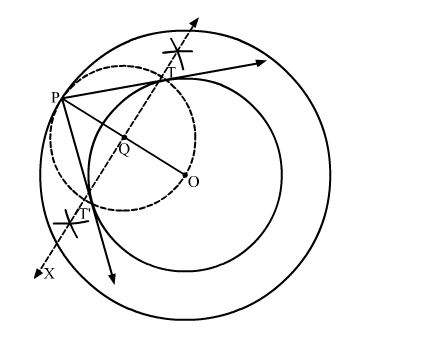# Construct a tangent to a circle of radius 4 cm from a point on the concentric circle of radius 6 cm and measure its length.`
Question:

Construct a tangent to a circle of radius 4 cm from a point on the concentric circle of radius 6 cm and measure its length. Also, verify the measurement by actual calculation.

Solution:

Steps of Construction

Step 1. Mark a point O on the paper.

Step 2. With O as centre and radii 4 cm and 6 cm, draw two concentric circles.

Step 3. Mark a point P on the outer circle.

Step 4. Join OP.

Step 5. Draw the perpendicular bisector XY of OP, cutting OP at Q.

Step 6. Draw a circle with Q as centre and radius OQ (or PQ), to intersect the inner circle in points T and T'.

Step 7. Join PT and PT'.Here, PT and PT' are the required tangents.

PT = PT' = 4.5 cm (Approx)

Verification by actual calculation

Join OT to form a right ∆OTP.     (Radius is perpendicular to the tangent at the point of contact)

In right ∆OTP,

$\mathrm{OP}^{2}=\mathrm{OT}^{2}+\mathrm{PT}^{2} \quad$ (Pythagoras Theorem)

$\Rightarrow \mathrm{PT}=\sqrt{\mathrm{OP}^{2}-\mathrm{OT}^{2}}$

$\Rightarrow \mathrm{PT}=\sqrt{6^{2}-4^{2}}=\sqrt{36-16}=\sqrt{20} \approx 4.5 \mathrm{~cm} \quad(\mathrm{OP}=6 \mathrm{~cm}$ and $\mathrm{OT}=4 \mathrm{~cm})$